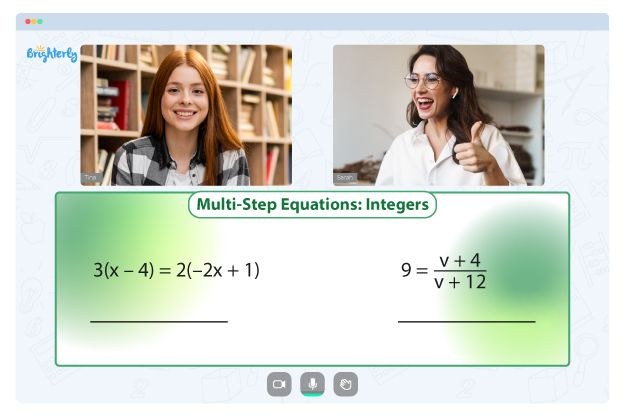# Logarithmic Worksheets

Logarithmic worksheets are a valuable resource for students who are learning about logarithms. A logarithm is a mathematical function that calculates the exponent required to produce a given number, often expressed in terms of base 10 or base e.

### What Are Logarithms Worksheets?

A logarithms worksheet is a set of problems and exercises designed to help students understand the principles and applications of logarithms. These worksheets typically cover a range of topics, including logarithm rules and properties, logarithmic equations, and logarithmic functions.

Math for Kids

Is Your Child Struggling With Math?
1:1 Online Math TutoringThe logarithm worksheet with answers PDF provides students with immediate feedback on their progress and helps them identify areas where they need further practice. There is also the log properties worksheet which focuses on the rules and properties of logarithms, including the product rule, quotient rule, and power rule.

### Benefits of Logarithm Worksheets

Our logarithm worksheet is a structured and comprehensive approach to learning about logarithms. These worksheets allow students to practice their skills and build their confidence as they work through problems at their own pace. Moreover, logarithmic worksheets can help students understand the practical applications of logarithms, such as the measurement of sound intensity and earthquake magnitude.

Whether you’re looking for a logarithm worksheet, log worksheet, or log properties worksheet, you can find them at Brighterly. Download our logarithm worksheet to help your kids improve their understanding of this important mathematical concept and develop their problem-solving skills.### Log Worksheet PDF

Log Worksheet### Logarithm Worksheet with Answers PDF### Logarithm Worksheet PDF

Logarithm Worksheet### Logarithms Worksheet PDF

Logarithms Worksheet

Need help with Equations?• Start studying with an online tutor.

Is your child finding it challenging to understand equations? An online tutor could be the solution.